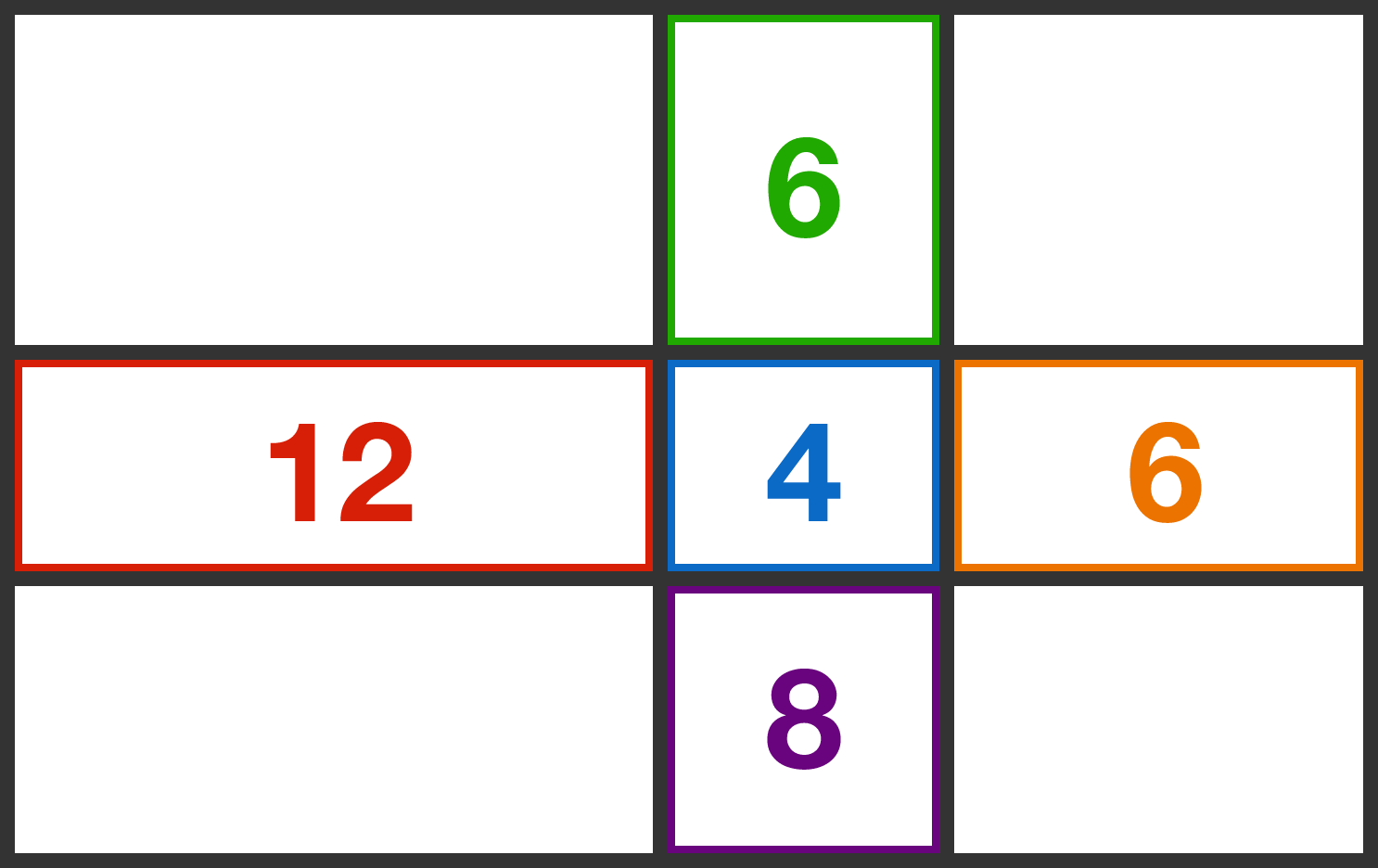# Problems of the Week

Contribute a problem

# 2017-11-06 Basic

In an office with 4 people, the 1 manager always lies and the 3 engineers always tell the truth. Who is the manager?Alice: "Bob is an engineer."
Bob: "Alice is an engineer."

Carol: "David is the manager."
David: "Carol is the manager."

Alice: "David is the manager."
David: "Alice is the manager."

True or False?

$\large 1\div\Big(2\div (3 \div (4\div ( \cdots \div (2015\div (2016\div 2017) ) \cdots \Big) > 1$

$1$ is an $\color{#D61F06}\text{odd}$ number.
$1 + 2$ is an $\color{#D61F06}\text{odd}$ number.
$1 + 2 + 3$ is an $\color{#3D99F6}\text{even}$ number.
$1 + 2 + 3+ 4$ is an $\color{#3D99F6}\text{even}$ number.
$1 + 2 + 3+ 4+5$ is an $\color{#D61F06}\text{odd}$ number.
$\cdots \cdots$

True or False?

$1 + 2 + 3 + \cdots + 999$ is an $\color{#D61F06}\text{odd}$ number.A rectangle is divided into nine sub-rectangles, as shown in the figure. Inside some rectangles are their respective perimeters.

What is the perimeter of the large, outer rectangle?Sara is returning home from a birthday party in a van. She has a helium balloon tied to the seat in front of her. If the van driver suddenly steps on the brake pedal very hard, what will happen to Sara and her balloon, relative to the van?

×